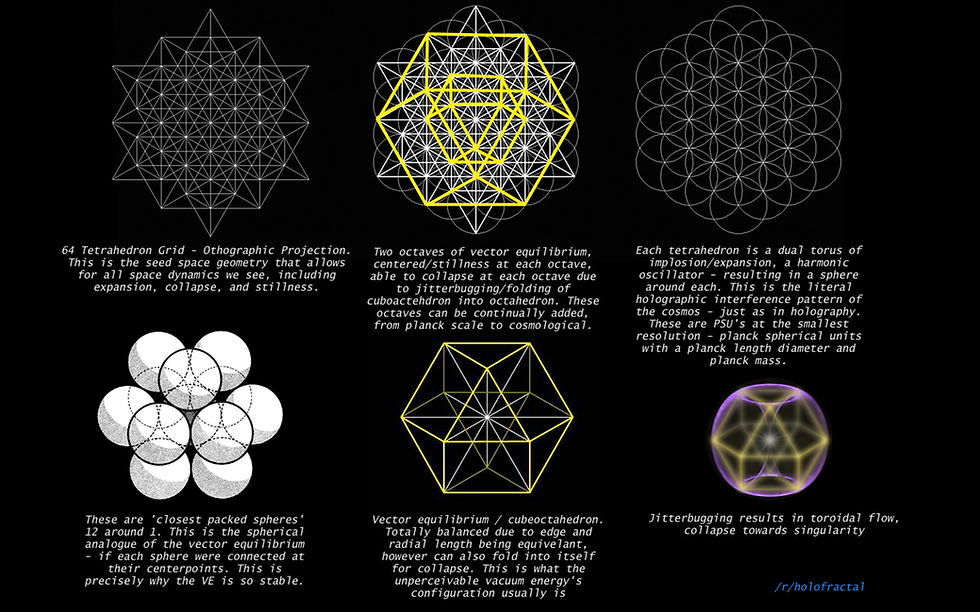top of page
Search

# tree of life superstring theory part 27

Updated: Sep 27, 2020numbers up to 64 and the numbers related to 3,6 and 9 on the tree of life and 6 the universe and 5 the atoms and strings on the tree of life and 8 tree of life's make the 64 tetrahedron grid(E8 lie group) the tetragrammaton is the leters of god and is the isotropic vector matrix which is 1,2,3 and 4 each leter has a mathematical number atached to it the numbers are 10,15,21 and 26 they all add up to 72 and 2(tetragrammaton)=144=(64 tetrahedron grid(E8 lie group)) 10 yods (spheres) that are joined by pathways of creation which is the tree of life the yods are connected to a crown yod by a root and in the crown yod god goes to infinity(9xen) so (tree of life)=9 yods and (8 tree of life's)=(64 tetrahedron grid) 72=9 144=9